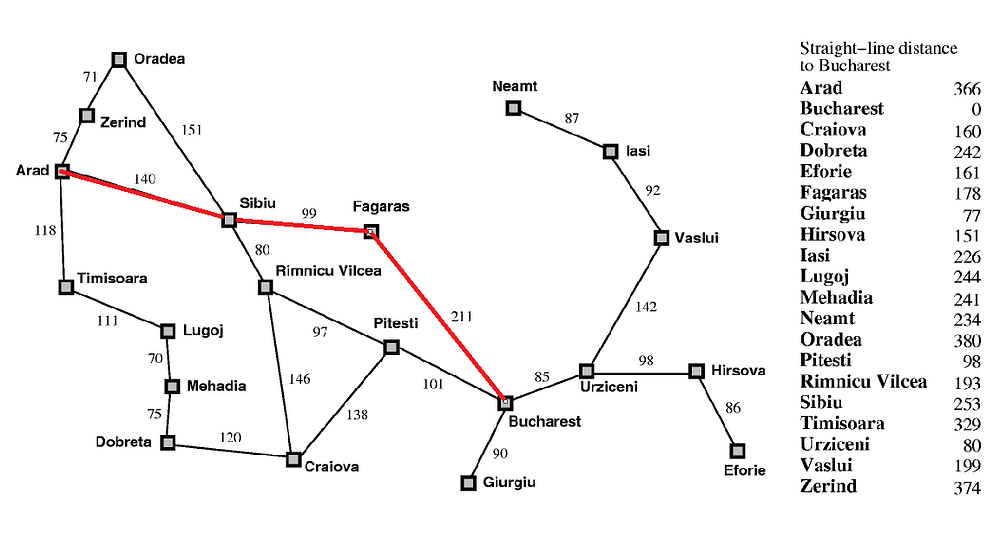# A* Algorithm in Artificial Intelligence

Original article was published on Artificial Intelligence on Medium# A* Algorithm in Artificial Intelligence

The environment we face everyday sends us an indefinite amount of information through our senses. It is a problem of cognition to be able to select the relevant information. If we want an artificially intelligent agent it must require the ability to guide itself through and find the relevant information. In A.I this is referred to as a heuristic.

# “A heuristic function maps a state onto an estimate of the cost to the goal.”

In formalizing the heuristic search problem we arrive at:

If h(n1)<h(n2)

We guess that it is cheaper to get to the goal from n1 than from n2.

Where:

• h represents a heuristic
• We require that h(n)=0 for every node n whose terminal state satisfies the goal.
• Zero cost of achieving the goal from node that already satisfies the goal

Before we get into A*, let us review a motivating heuristic that inspires A*..

# Best First Search

Take a path from Arad to Bucharest by calculating the shortest path between nodes: